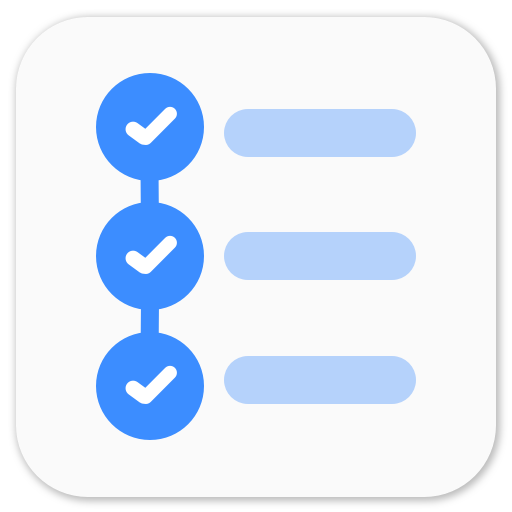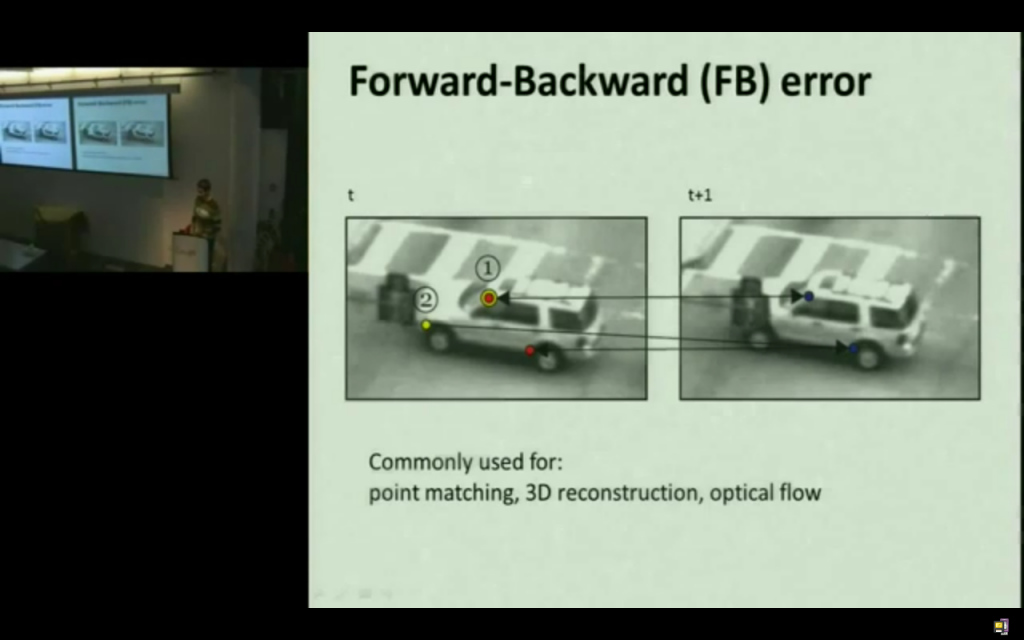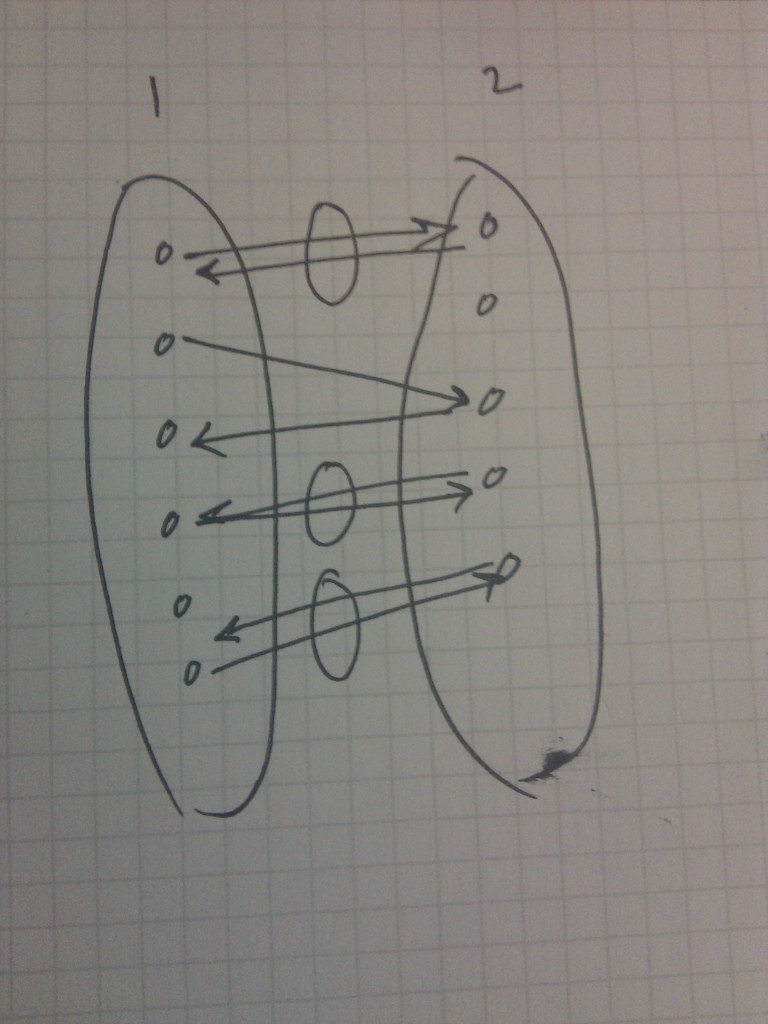Taskito - Calendar based to-do listI am working on a tracking algorithm based on Lucas-Kanade Method using Optical Flow. The Lucas–Kanade method is a widely used differential method for optical flow estimation developed by Bruce D. Lucas and Takeo Kanade. It assumes that the flow is essentially constant in a local neighbourhood of the pixel under consideration, and solves the basic optical flow equations for all the pixels in that neighbourhood, by the least squares criterion.

This method is less sensitive to image noise. This method assumes that the displacement of the object in the image between two consecutive frames is small and approximately constant within a neighborhood of the considered point. Thus the optical flow equation can be assumed to hold for all pixels within a window centered at the considered point. I am obtaining these points by using OpenCV’s goodFeaturesToTrack function within the provided bounding box.

After getting the points(good features to track), I am using OpenCV’s calcOpticalFlowPyrLK to get the corresponding features/points in the current frame using Lucas Kanade method based on Optical Flow using itrative pyramids. I am using Forward-Backward Error method to eliminate false positives. This is how Forward-Backward Error method works.

I have 2 consecutive image frames. There are m tracked points in the first image. Using some method(here Lucas Kanade mehotd with using Optical Flow), I determine the tracking points in the next image. I get n number of tracked points. This implies that there are some false positives. so, now I’ll use the same method on the second image to determin the tracking poitns in the first image. I will get some k numbers of tracking points. The common points in the m and k points would give the correct corresponding tracking points in the second image.After getting the tracked points, I am trying to predict the new bounding box in a very crude manner. I am working on it for improvements.

So, here’s the code of the tracker in OpenCV.

import cv2
cam = cv2.VideoCapture(0)
oldg = cv2.cvtColor(img, cv2.cv.CV_BGR2GRAY)

Setting the parameters.

lk_params = dict( winSize  = (10, 10),
maxLevel = 5,
criteria = (cv2.TERM_CRITERIA_EPS | cv2.TERM_CRITERIA_COUNT, 10, 0.03))

feature_params = dict( maxCorners = 3000,
qualityLevel = 0.5,
minDistance = 3,
blockSize = 3)

Get the good features to track.

img1 = img[bb:bb,bb:bb] # crop the bounding box area
g = cv2.cvtColor(img1, cv2.cv.CV_BGR2GRAY) # get grayscale image
pt = cv2.goodFeaturesToTrack(g, **feature_params)
# pt is for cropped image. add x, y in each point.
for i in xrange(len(pt)):
pt[i] = pt[i]+bb
pt[i] = pt[i]+bb

Apply Lucas Kanade method with Optical Flow

p0 = np.float32(pt).reshape(-1, 1, 2)
newg = cv2.cvtColor(img, cv2.cv.CV_BGR2GRAY)
p0 = np.float32(pt).reshape(-1, 1, 2)

# For Forward-Backward Error method
# using calcOpticalFlowPyrLK on both image frames
# with corresponding tracking points

p1, st, err = cv2.calcOpticalFlowPyrLK(oldg, newg, p0,
None, **lk_params)
p0r, st, err = cv2.calcOpticalFlowPyrLK(newg, oldg, p1,
None, **lk_params)
d = abs(p0-p0r).reshape(-1, 2).max(-1)
good = d < 1
new_pts = []
for pts, val in itertools.izip(p1, good):
if val:
# points using forward-backward error
new_pts.append([pts, pts])

After getting the tracking points, you might predict the new bounding box using some algorithm.

Here’s the video of the Lucas Kanade Tracker that I implemented in SimpleCV using OpenCV (The above code).

Here’s the complete LK-OpenCV code.

P.S. GSoC is about to end and I’m back to the campus.

Playing around with Android UI

Articles focusing on Android UI - playing around with ViewPagers, CoordinatorLayout, meaningful motions and animations, implementing difficult customized views, etc.